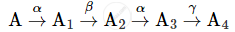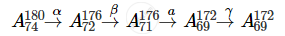# A Radioactive Nucleus 'A' Undergoes a Series of Decays as Given Below: - Physics

A radioactive nucleus 'A' undergoes a series of decays as given below:The mass number and atomic number of A2 are 176 and 71 respectively. Determine the mass and atomic numbers of A4 and A.

#### SolutionThe mass number and atomic number of A4 is 172 and 69, respectively.

The mass number and atomic number of A is 180 and 74, respectively.

Is there an error in this question or solution?
2016-2017 (March) Delhi Set 1

Share